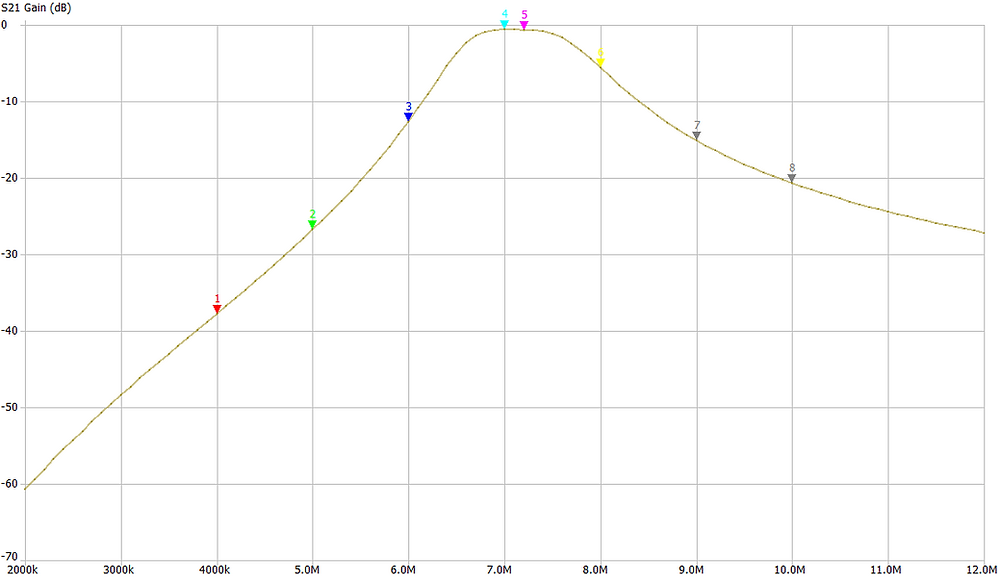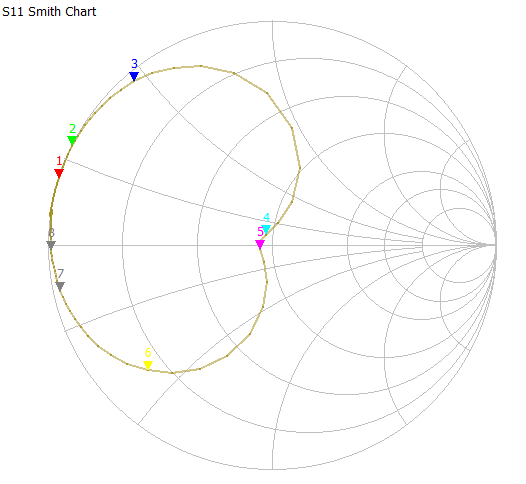Search
• xtgaby

# Bandpassfilter for 40 meter band (7MHz) with Amidon T50-2 cores.

Updated: Nov 27, 2020

## Picture:The Kank 3334 transformer from Toko has on one (primary) side 18 turns (5.5 uH) and on the other (secundary) side 3 turns ==> this means a ratio of 18/3 = 6..

The T50-2 core from amidon (50 stands for 0.50 inch and 2 stands for the material) has an AL value of 49 uH.

Al value means that when you use 100 turns on the core, the coil wil have a value of 49 uH.

Material 2 is ideal for the use between 250 KHz and 10 MHz.

Calculation how many turns are required.

- Now we know that to make a coil of 49 uH, we need to have 100 turns on our core.

- Our needed value is 5.5 uH to have the same value as the Toko coil.

- 49 uH/5.5uH = 8.9

- Coil value is dependant of the square of the number of turns.

- 100 x 100 = 10.000 / 8.9 = 1123. Root of 1123 = 34 turns required for 5.5uH.

- number of secundary turns = 34 / 6 (ratio) = approx. 6 turns required.

Calculation value of de capacitor required.

Resonance frequency wanted = 7.1MHz## Schematic: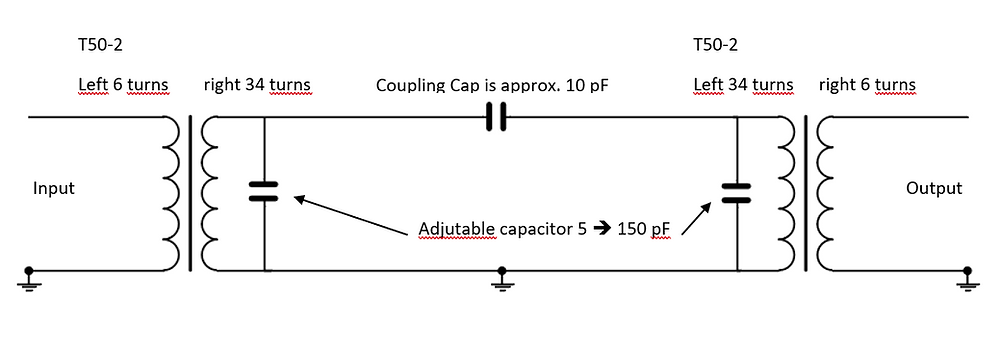## Results: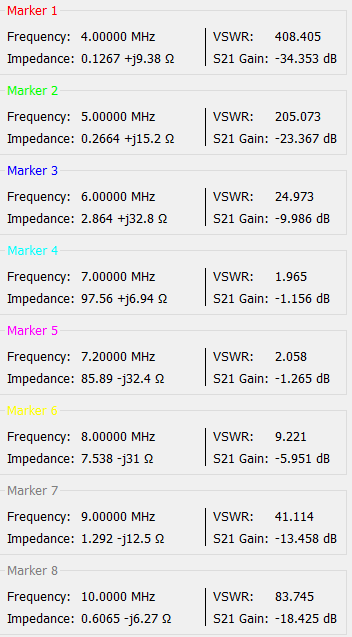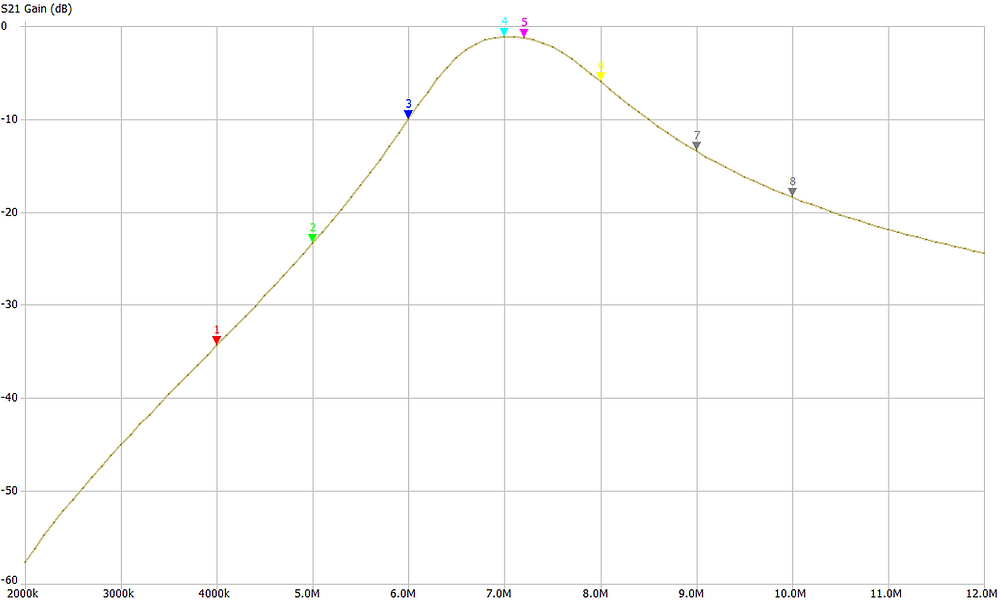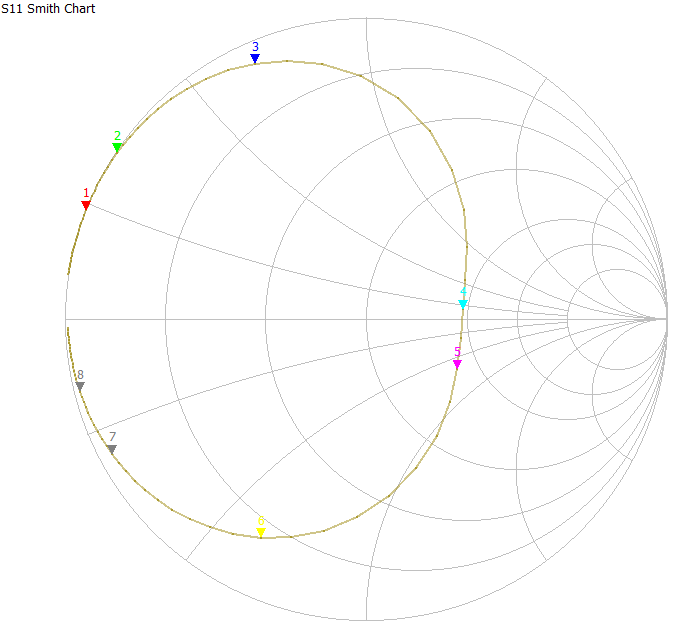## Due to the fact that SWR was 2 at 7 MHz (about 100 Ohms), I have changed the numer of turns from 6 to 5. This gives a much better SWR and a better insertion loss.## The new results: